# Wrapture

Computer-generated audio/visual work
Lance Putnam (2007), 8 min 27 sec
Video (360p)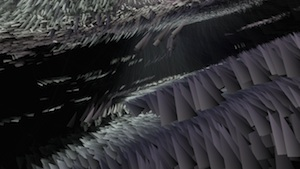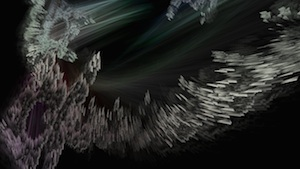## Summary

Wrapture is a passage through an abstract mathematical space based on a 4-dimensional iterated map. Periodic boundaries are used to introduce non-linearity and strange attractors into the system. This rupture of order serves to provide an underlying structure for sonic and visual forms. The iterated map, while only producing a one-dimensional orbit, manifests a vast range of complex whirlpools, galaxies and constellations.

## Details

The mathematical system used is an iterated function that produces a sequence of 4-dimensional coordinates called an orbit. The function is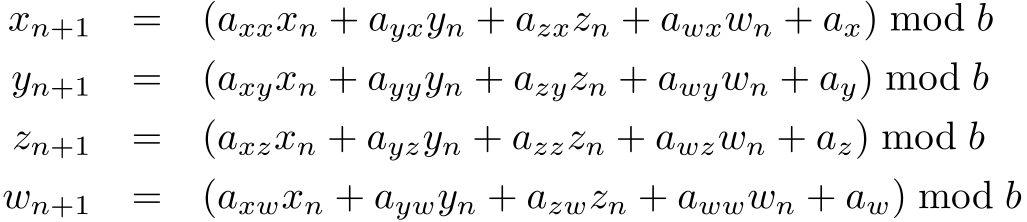This system can be dissected into both a linear and non-linear part. The linear part is simply a recursive application of a transformation matrix on a 4-vector (a coordinate of the orbit). In terms of matrix multiplication, the linear part can be written asThose versed in computer graphics should recognize this as a 5-dimensional homogenous coordinate transformation. The matrix represents a scaling, rotation, and translation in four dimensions. Recursively applied, this transformation matrix will result in a spiraling orbit.

The non-linear part is the application of a modulo operation. This forces what was previously an unbounded Euclidean space to be wrapped into a bounded toroidal topology. Not only does the modulo operation constrain the orbit to a finite region of space, but it also adds a considerable degree of chaotic behavior to the system. The system will remain linear as long as the determinant of the matrix in the linear part remains less than the modulo amount.

The orbit is plotted spatially using its x, y, and z components and the w component determines the orientation of a small triangular glyph. The three angles made by the vectors (w,x), (w,y), and (w,z) are used as Euler angles to rotate the triangle. As a result of this mapping, the forms have both an extended form due to spatial coordinates (x,y,z) and a texture due to an internal property (w). The w component also determines the hue of each glyph. The spatiotemporal orbit is mapped into sound by defining a spectral mask on a complex chord of saw waves. The mask is constructed by adding together histograms of points along the orbit for each dimension.

## "Version 1"

The most current version of Wrapture stemmed from an earlier work I did in 2007. This version was entirely grayscale and used only simple points to display each output coordinate of the equation. The manner in which the fourth dimension was represented was also quite different. Instead of rendering a triangle glyph at each point oriented according to the fourth dimension, this version simultaneously displayed multiple 3-dimensional projections of the 4-dimensional space. This technique is analogous to looking at the shadows cast by a 3-dimensional object on 3 mutually perpendicular walls (as exemplified here). Below are some images of piece where these multiple projections can be seen.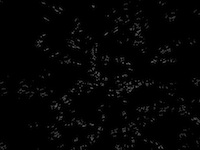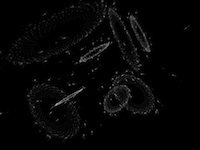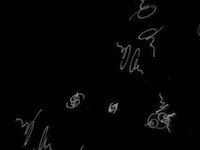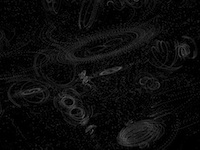A video (super low-res) can be found here.